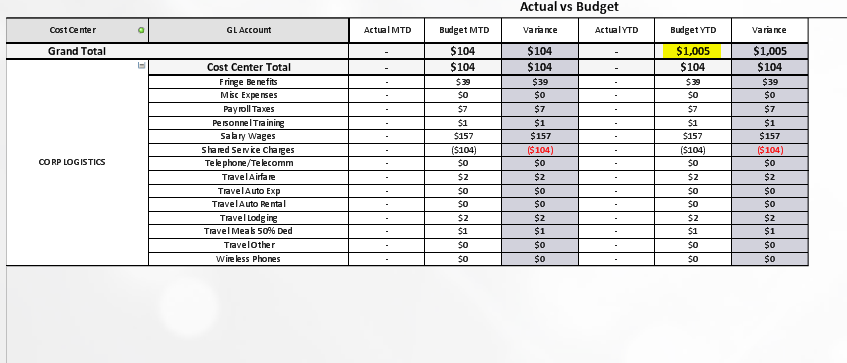# QlikView App Development

Discussion Board for collaboration related to QlikView App Development.

Announcements
Leverage your QlikView investment to modernize BI – see how! Join Group
cancel
Showing results for
Did you mean:
HighlightedContributor

## Pivot Table Totals

I have a pivot table that calculates MTD and YTD. The problem is that the expression total (for YTD) does not respond to selections. For example, when I select January the Grand totals for MTD and YTD are calculating correctly, but when I select a cost center, the MTD will adjust, but the YTD will be frozen. See screen shots below.

The YTD formula I am using is sum( {1<[CLMonthID] = {"<=\$(vCLMonthID)"} >}  [CL B2017])2 Replies
HighlightedContributor III

## Re: Pivot Table Totals

Try changing the formula into:

sum( {\$<[CLMonthID] = {"<=\$(vCLMonthID)"} >}  [CL B2017])

HighlightedMVP

## Re: Pivot Table Totals

Why do you have 1 in your set expression?

Sum({1<[CLMonthID] = {"<=\$(vCLMonthID)"}>}  [CL B2017])

May be try without it?

Sum({<[CLMonthID] = {"<=\$(vCLMonthID)"}>}  [CL B2017])

or allow for selection selection in Cost Center

Sum({1<[CLMonthID] = {"<=\$(vCLMonthID)"}, [Cost Center] = \$::[Cost Center]>}  [CL B2017])